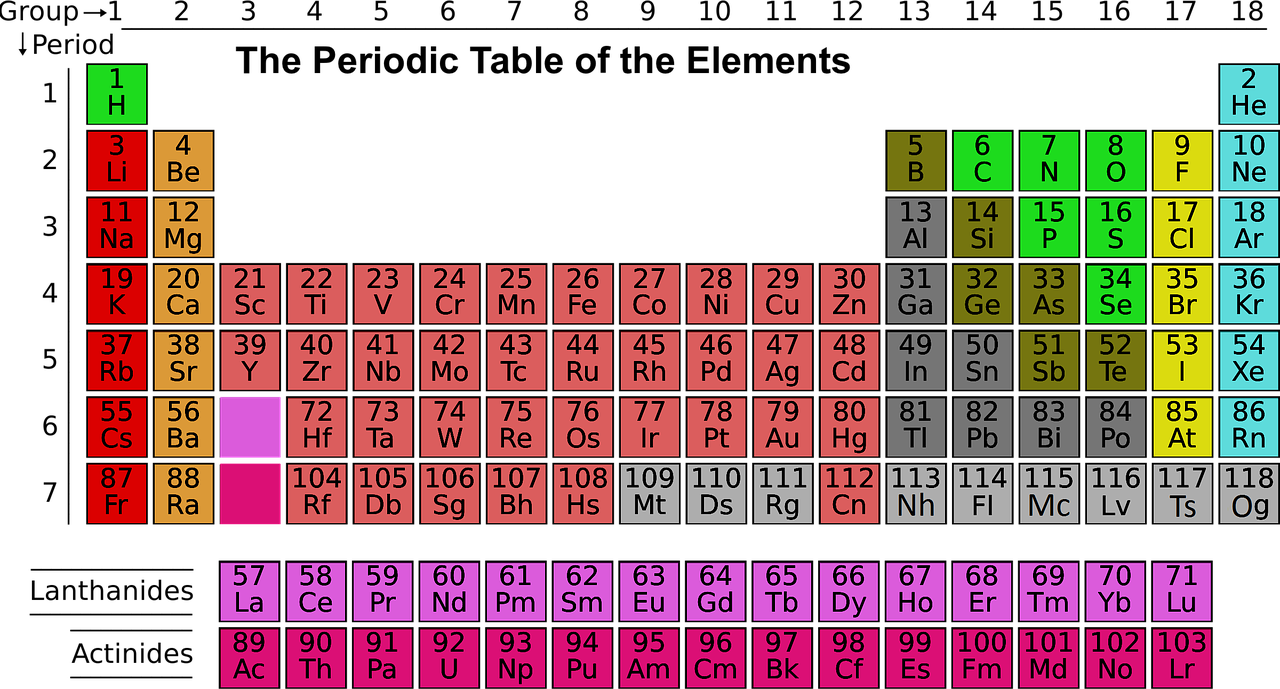Courses
Courses for Kids
Free study material
Offline Centres
MoreLast updated date: 27th Nov 2023
Total views: 306k
Views today: 7.06k

# How many electrons are in the highest occupied energy level of a group $5A$ element?Verified
306k+ views
Hint: The Group $5A$ elements have five valence electrons in their most elevated energy orbital$\left( {n{s^2}n{p^3}} \right)$ . Nitrogen, phosphorus, and arsenic can frame ionic compounds by acquiring three electrons, shaping the nitride$\left( {{N^{3 - }}} \right)$ , phosphide $\left( {{P^{3 - }}} \right)$ and arsenide $\left( {A{s^{3 - }}} \right)$ anions, however they all the more as often as possible structure compounds through covalent holding. Antimony and bismuth can lose either their outermost $p$ electrons to shape $3 +$ charges, or their outermost $s$ and $p$ electrons to frame $5 +$ charges.

The thing to recall about a main-group element is that the group number discloses to you the number of valence electrons said component has.Principal bunch components are situated in groups$1$,$2$ and $13$ through $18$ . For your situation, the group number is given using the old notation that utilizes Roman numerals to recognize primary groups, $A$ , and transition components,$B$ .
You thus have:
Group1 Group1A
Group2 Group2A
Group13 Group13A
Group14 Group14A
Group15 Group15A
And so on
Presently, your component is supposed to be situated in group$5A$ , for example group$15$ . For this group, the quantity of valence electrons is recorded in the group number
Group5A valence electrons
Group15A valence electrons
As you probably are aware, an element's valence electrons are simply the electrons situated on the outermost energy level, for example on the highest occupied energy level.
In this way, you can say that element situated in group $5A$ has $5$ electrons on its highest occupied energy level.
Note: The general formula is that the nth shell can on a fundamental level hold up to $2\left( {{n^2}} \right)$ electrons. Since electrons are electrically attracted to the nucleus, an atom's electrons will for the most part involve external shells just if the more internal shells have just been totally filled by different electrons.